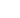#Management Accounting: Decision and Control Test Two Q1 of 15

The following information has been calculated for the production of 1 unit of Flotsam

• Each unit will require 1.55 kilograms of material at a total cost of £31.00
• Each unit will require 75 minutes of labour at a cost of £16.00 per hour
• Fixed overheads total £60,000 and the estimated output will be 12,000 units of Flotsam
• Fixed overheads are absorbed on a labour hour basis

Complete the standard cost card below. Show all figures to 2 decimal places.

 1 unit of Flotsam Quantity Cost per unit £ Total cost £ Material kgLabour hoursFixed costs hoursTotal